• 图像锐化演示-图像锐化.rar 自己编写的教学演示程序。 内容图像锐化包括梯度法、sobel算子、laplacian算子、prewitt算子和高通滤波。 由于权限不够，能发图片，演示效果截图在附件中。matlab
• ## 图像增强—图像锐化

万次阅读 多人点赞 2019-08-09 13:16:32
图像锐化与图像平滑是相反的操作，锐化是通过增强高频分量来减少图像中的模糊，增强图像细节边缘和轮廓，增强灰度反差，便于后期对目标的识别和处理。锐化处理在增强图像边缘的同时也增加了图像的噪声。方法通常有...
文章目录1. 图像锐化1.1. 图像细节的灰度变化特性2. 锐化处理方法2.1. 微分法2.1.1. 梯度法2.1.1.1. 直接以梯度代替锐化输出2.1.1.2. 输出阈值判断2.1.1.3. 为边缘规定一个特定的灰度级2.1.1.4. 为背景规定特定的灰度级2.1.1.5. 二值化图像2.2. 罗伯特梯度算子法2.3. 拉普拉斯算子法2.4. 高通滤波法2.5. 模板匹配法2.5.1. Sobel算子2.5.2. Prewitt算子3. 一些边缘检测的经验
1. 图像锐化
图像锐化与图像平滑是相反的操作，锐化是通过增强高频分量来减少图像中的模糊，增强图像细节边缘和轮廓，增强灰度反差，便于后期对目标的识别和处理。锐化处理在增强图像边缘的同时也增加了图像的噪声。方法通常有微分法和高通滤波法。
1.1. 图像细节的灰度变化特性
取图像中的某一行作为扫描行，其灰度变化曲线为：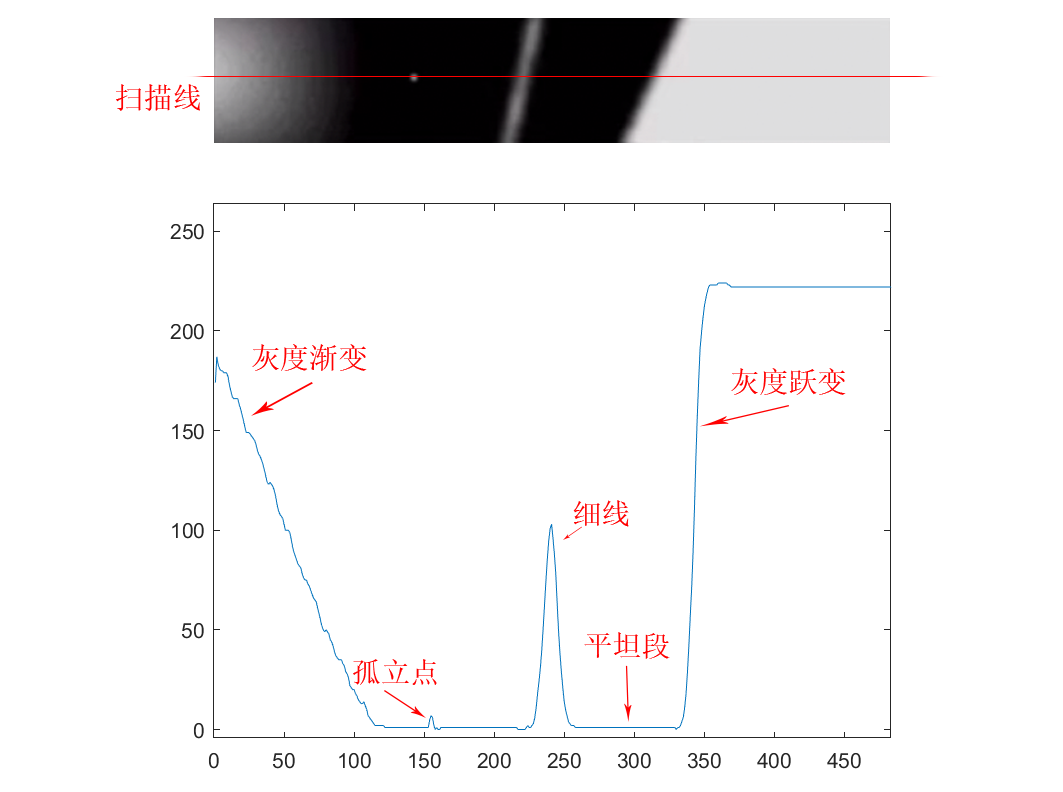对该灰度变化曲线进行微分，得到一阶微分曲线和二阶微分曲线：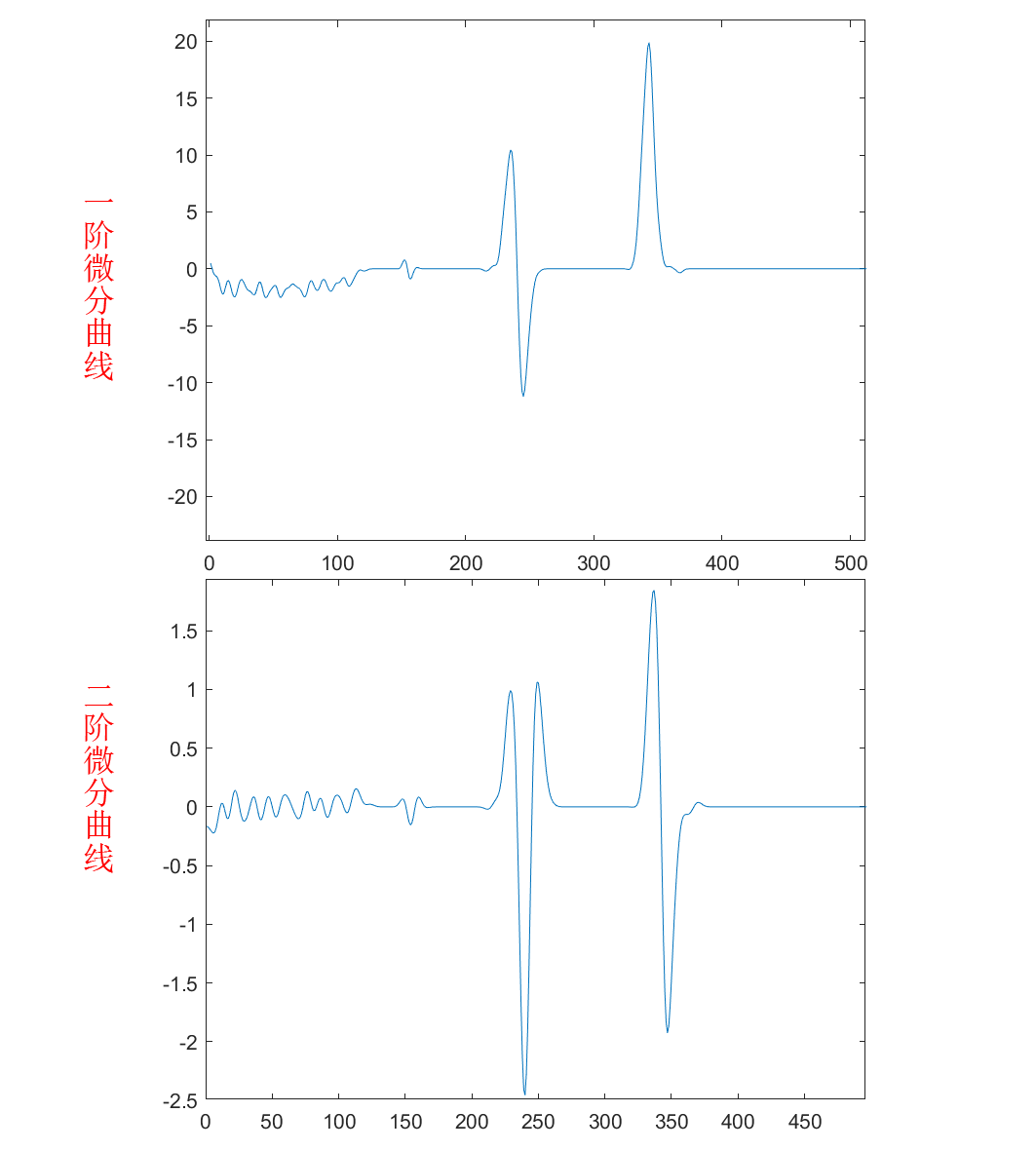由图像的灰度变化曲线及其一阶微分和二阶微分可以总结出三种典型的灰度截面特征

阶跃型
细线型
斜坡渐变型

灰度变化曲线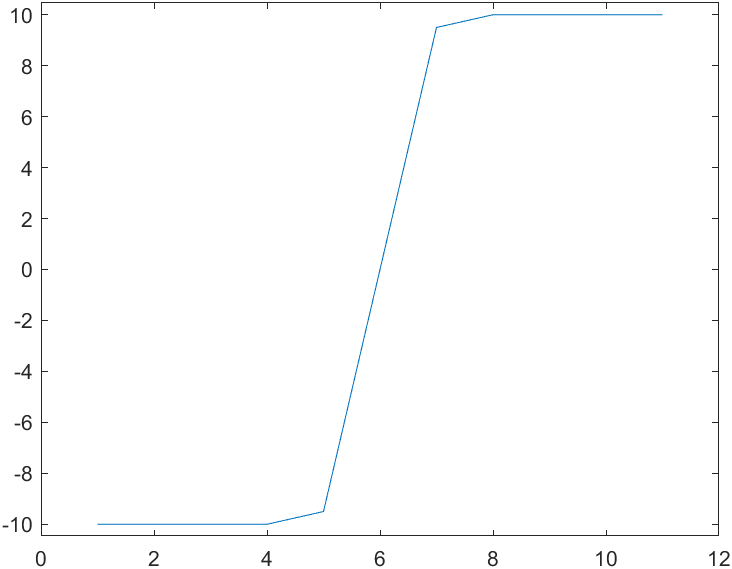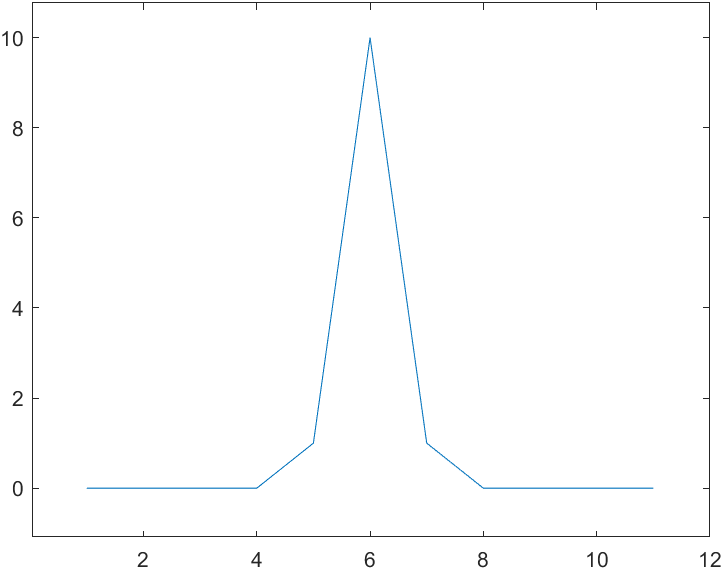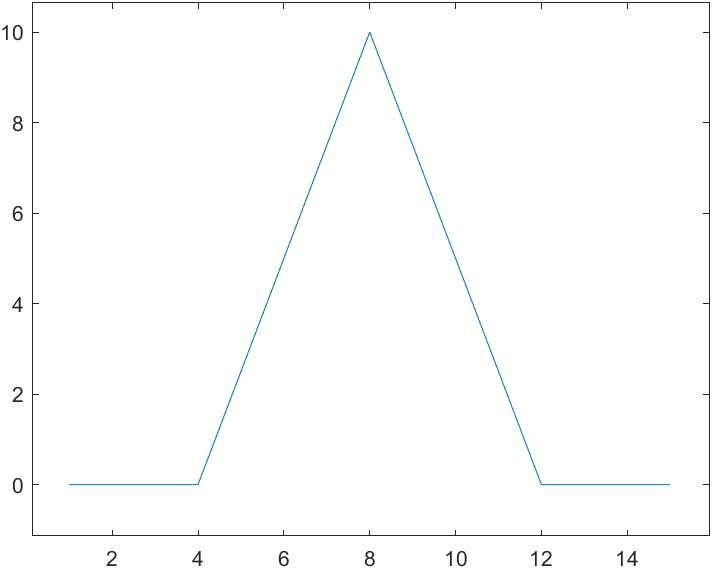一阶微分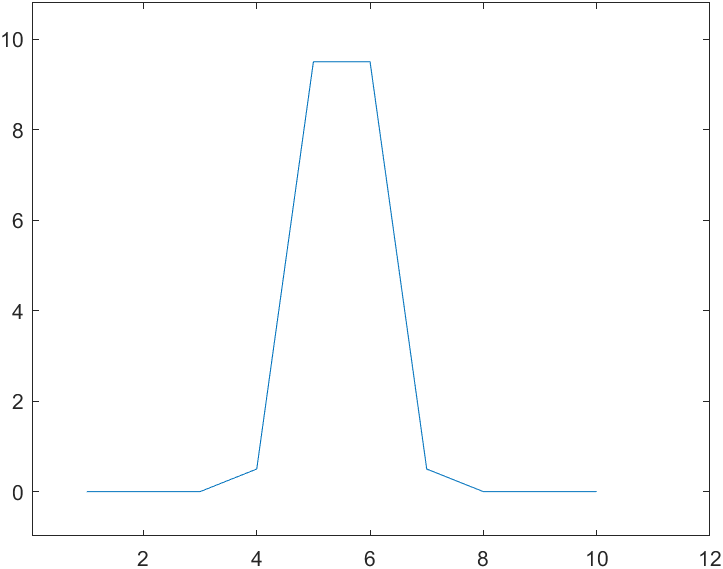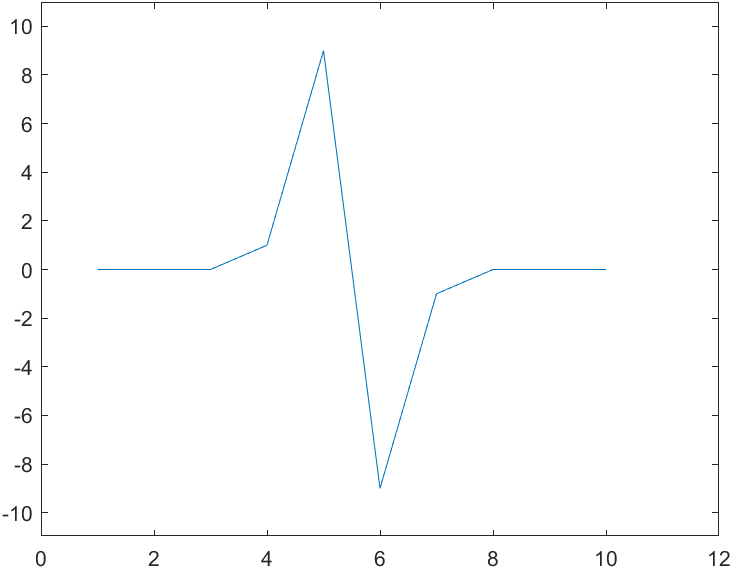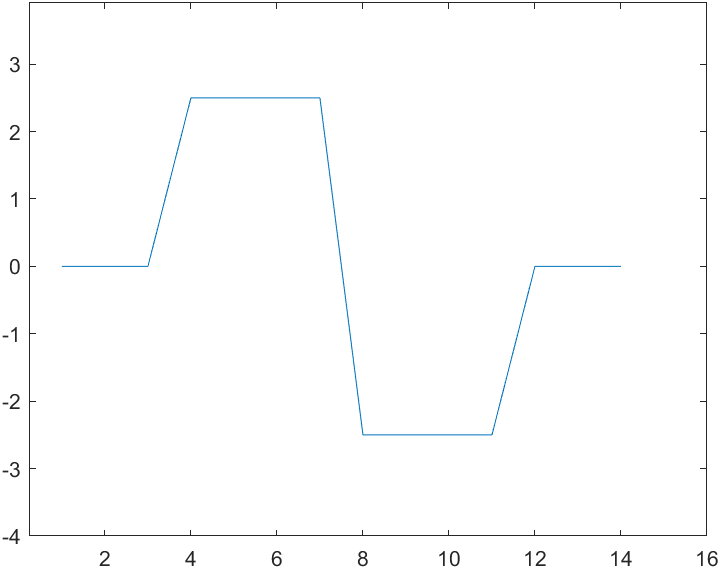二阶微分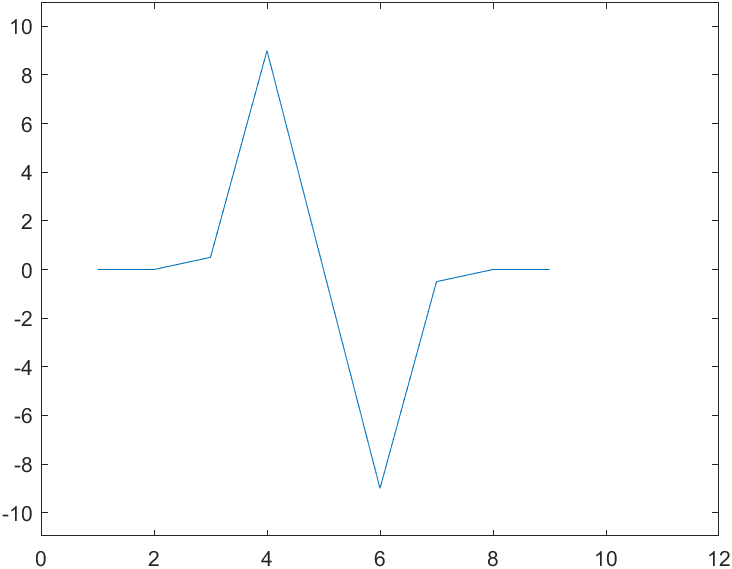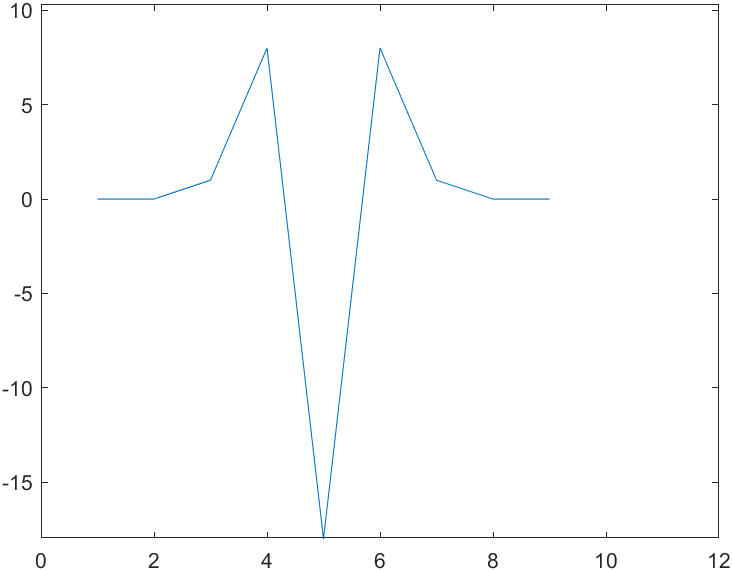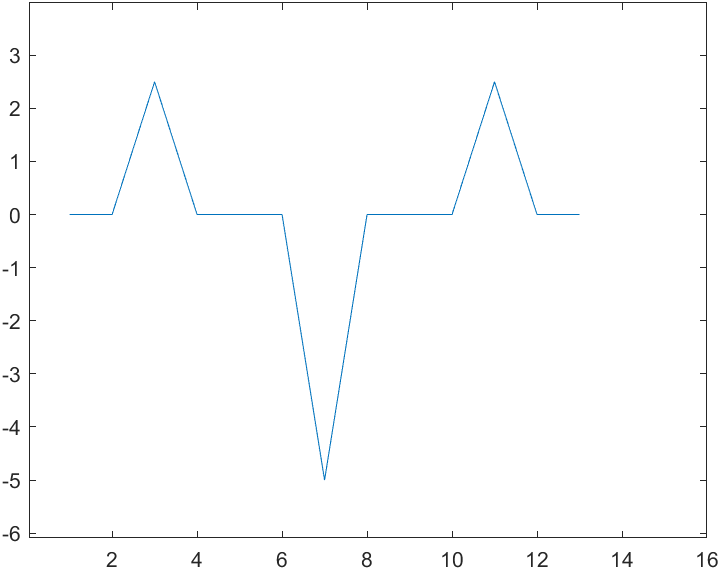2. 锐化处理方法
2.1. 微分法
2.1.1. 梯度法
梯度的方向是图像中最大变化率的方向，梯度的幅度比例于相邻像素的灰度级差值
对图像$F(x,y)$，在点$(x,y)$处的梯度定义为矢量：$\nabla F(x,y)=\begin{bmatrix} \frac{\partial F}{\partial x} \\ \\ \frac{\partial F}{\partial y} \\ \end{bmatrix}$其幅度(模)为：$|\ \nabla F(x,y)\ |=\sqrt{( \frac{\partial F}{\partial x})^2+( \frac{\partial F}{\partial y})^2}$在进行图像处理时所称的梯度通常是指梯度的模。
对于离散图像，一般用邻近相差的差分法来代替微分：\begin{aligned} \nabla F(x,y) & = \sqrt{|\ [F(x,y)-F(x+1, y)]^2 + [F(x,y)-F(x,y+1)]^2\ |} \\ & \approx |\ [F(x,y)-F(x+1, y)]\ | + |\ [F(x,y)-F(x,y+1)]^2\ | \\ \end{aligned}
有了梯度$\nabla F(x,y)$之后就可以根据梯度得到锐化结果(输出)，这里介绍五种确定锐化输出$G(x,y)$的方法
2.1.1.1. 直接以梯度代替锐化输出
$G(x,y)=\nabla F(x,y)$该方法简单，但是在图像均匀的区域由于梯度很小，会导致锐化输出图像整体偏暗
2.1.1.2. 输出阈值判断
$G(x,y) = \begin{cases} \nabla F(x,y), & \nabla F(x,y)>T \\ F(x,y), & otherwise \end{cases}$该方法不会破坏图像背景，同时又可以进行一定程度的图像锐化
2.1.1.3. 为边缘规定一个特定的灰度级
$G(x,y) = \begin{cases} L_\alpha, & \nabla F(x,y)>T \\ F(x,y), & otherwise \end{cases}$
2.1.1.4. 为背景规定特定的灰度级
$G(x,y) = \begin{cases} \nabla F(x,y), & \nabla F(x,y)>T \\ L_\beta, & otherwise \end{cases}$
2.1.1.5. 二值化图像
$G(x,y) = \begin{cases} L_\alpha, & \nabla F(x,y)>T \\ L_\beta, & otherwise \end{cases}$
2.2. 罗伯特梯度算子法
Roberts梯度算子法算法简单，无方向性，采用对角相差的差分法来代替微分：\begin{aligned} \nabla F(x,y) & = \sqrt{|\ [F(x,y)-F(x+1, y+1)]^2 + [F(x+1,y)-F(x,y+1)]^2\ |} \\ & \approx |\ [F(x,y)-F(x+1, y+1)]\ | + |\ [F(x+1,y)-F(x,y+1)]^2\ | \\ \end{aligned}
2.3. 拉普拉斯算子法
Laplacian算法是线性二次微分算子，与梯度算子一样具有旋转不变性，从而满足了不同方向的图像边缘锐化要求，其获得的边界比较细，包括较多的细节信息，但边界不清晰
Laplacian算子：$\nabla^2 F(x,y)=\frac{\partial^2 F(x,y)}{\partial x^2}+\frac{\partial^2 F(x,y)}{\partial y^2}$其离散形式为：\begin{aligned} \nabla^2 F(x,y) & = \{\ [F(x+1,y)-F(x,y)]-[F(x,y)-F(x-1,y)]\ \}+\{\ [F(x,y+1)-F(x,y)]-[F(x,y)-F(x,y-1)]\ \} \\ & = F(x+1,y)+F(x-1,y)+F(x,y+1)+F(x,y-1)-4F(x,y) \\ \end{aligned}可以看出，Laplacian算子就是其4倍中心元素值与其领域值和之差的绝对值，也可表达为中心元素与其领域内元素和均值负5倍的绝对值：$\nabla^2 F(x,y)=|-5\{\ F(x,y)-\frac{1}{5}[F(x-1, y)+F(x, y-1)+F(x,y)+F(x,y+1)+F(x+1,y+1)]\ \}|$
使用Laplacian算子进行锐化时，其锐化输出$G(x,y)$为：$G(x,y)=F(x,y)-\nabla^2F(x,y)$
常用的三种Laplacian算子：
$\begin{bmatrix} 0 & -1 & 0 \\ -1 & 4 & -1 \\ 0 & -1 & 0 \\ \end{bmatrix}\ \ \ \ \ \ \begin{bmatrix} -1 & -1 & -1 \\ -1 & 8 & -1 \\ -1 & -1 & -1 \\ \end{bmatrix}\ \ \ \ \ \ \begin{bmatrix} 1 & -2 & 1 \\ -2 & 4 & -2 \\ 1 & -2 & 1 \\ \end{bmatrix}$
在图像处理中，为了改善锐化的效果，也可以脱离微分计算原理，在原有算子的基础之上对模板系数进行改变，得到Laplacian变形算子
2.4. 高通滤波法
图像的边缘与频域中的高频分量相对应，高通滤波器可以抑制低频分量，从而达到图像锐化的目的
建立在离散卷积基础上的空域高通滤波关系式为：$g(\mu,\nu)=\sum_{x}^{}\sum_{y}^{}F(x,y)H(\mu-x+1,\nu-y+1)$其中$H(\mu-x+1,\nu-y+1)$被称作冲激响应
常用的五种归一化冲激响应阵列：
$H_1=\begin{bmatrix} 0 & -1 & 0 \\ -1 & 5 & -1 \\ 0 & -1 & 0 \\ \end{bmatrix}\ \ \ \ \ \ H_2=\begin{bmatrix} -1 & -1 & -1 \\ -1 & 9 & -1 \\ -1 & -1 & -1 \\ \end{bmatrix}\ \ \ \ \ \ H_3=\begin{bmatrix} 1 & -2 & 1 \\ -2 & 5 & -2 \\ 1 & -2 & 1 \\ \end{bmatrix}$$H_4=\frac{1}{7}\begin{bmatrix} 0 & -2 & 0 \\ -2 & 19 & -2 \\ 0 & -2 & 0 \\ \end{bmatrix}\ \ \ \ \ \ H_5=\frac{1}{2}\begin{bmatrix} -2 & -1 & -2 \\ -1 & 6 & -1 \\ -2 & -1 & -2 \\ \end{bmatrix}$
2.5. 模板匹配法
将具有8个方向、大小为3×3的模板顺序作用于同一图像窗口，并对结果进行排序，将最大的结果值会加权平均值作为窗口中心元素的锐化进行输出。
模板匹配法除了能增强图像边缘外还具有平滑噪声的优点，总体上效果优于梯度法
下面介绍两种模板匹配算子：
2.5.1. Sobel算子Sobel算子能够锐化的边缘信息较强，其运算定义为：$g(x,y)=|V_x|+|V_y|$其中：
$V_x=[f(x-1,y-1)+2f(x-1,y)+f(x-1,y+1)]-[f(x+1,y-1)+2f(x+1,y)+f(x+1,y+1)]$$V_x=[f(x-1,y-1)+2f(x,y-1)+f(x+1,y-1)]-[f(x-1,y+1)+2f(x,y+1)+f(x+1,y+1)]$
2.5.2. Prewitt算子
Prewitt算子有一定的抗干扰性，图像效果比较干净。其有8个方向，分别对应8个模板：
$\uparrow：\begin{bmatrix} 1 & 1 & 1 \\ 1 & -2 & 1 \\ -1 & -1 & -1 \\ \end{bmatrix}\ \ \ \ \ \ \nearrow：\begin{bmatrix} 1 & 1 & 1 \\ -1 & -2 & 1 \\ -1 & -1 & 1 \\ \end{bmatrix}\ \ \ \ \ \ \rightarrow：\begin{bmatrix} -1 & 1 & 1 \\ -1 & -2 & 1 \\ -1 & 1 & 1 \\ \end{bmatrix}\ \ \ \ \ \ \searrow：\begin{bmatrix} -1 & -1 & 1 \\ -1 & -2 & 1 \\ 1 & 1 & 1 \\ \end{bmatrix}$$\downarrow：\begin{bmatrix} -1 & -1 & -1 \\ 1 & -2 & 1 \\ 1 & 1 & 1 \\ \end{bmatrix}\ \ \ \ \ \ \swarrow：\begin{bmatrix} 1 & -1 & -1 \\ 1 & -2 & -1 \\ 1 & 1 & 1 \\ \end{bmatrix}\ \ \ \ \ \ \leftarrow：\begin{bmatrix} 1 & 1 & -1 \\ 1 & -2 & -1 \\ 1 & 1 & -1 \\ \end{bmatrix}\ \ \ \ \ \ \nwarrow：\begin{bmatrix} 1 & 1 & 1 \\ 1 & -2 & -1 \\ 1 & -1 & -1 \\ \end{bmatrix}$
3. 一些边缘检测的经验

突变型细节，通过一阶微分的极大值点，二阶微分的零点可以检测出来
细线型细节，通过一阶微分的零点，二阶微分的极小值点可以检测出来
渐变型细节，一般情况下突变幅度小、定位难，不易检测，但二阶微分的信息比一阶微分多，需进行综合考虑

边缘信息具有方向性，不同的锐化/边缘检测算子也可能具有方向性，因此同一个算子对不同的图像检测的效果可能不同，应对不同图像使用不同的算子进行检测


展开全文高通滤波器 拉普拉斯算子
• 数字图像处理的，图像锐化包括Laplacian，Priwitt，Sobel，水平方向锐化。比较基础的，C写的
• 开发环境为QT5.8+opencv3.2，主要实现了图像增强，包括高斯平滑，中值滤波，图像锐化
• 数字图像锐化算法研究 图像锐化(2010-08-03 16:10:31)转载▼标签： 杂谈 分类： Matlab MATLAB全称是MatrixLaboratory(矩阵实验室)，一开始它是一种专门用于矩阵数值计算的软件，从这一点上也可以看出，它在矩阵...
• 图像锐化，是使图像边缘更清晰的一种图像处理方法，细节增强（detail enhancement）我理解也包含了图像锐化常用的做法是提取图像的高频分量，将其叠加到原图上。图像高频分量的提取有两种做法，一种是用高通滤波器...
图像锐化，是使图像边缘更清晰的一种图像处理方法，细节增强（detail enhancement）我理解也包含了图像锐化，常用的做法是提取图像的高频分量，将其叠加到原图上。图像高频分量的提取有两种做法，一种是用高通滤波器，得到高频分量，另一种是通过低通滤波，用原图减低频得以高频。
直接提取高频的方法有sobel算法、laplcian算子，sobel算子是图像的一阶导数，提取的是梯度信息，分水平和垂直两种，常常用来做边缘检测、方向判别，sobel算子在斜坡处不为0，因此会产生较粗的边缘。laplcian算子是图像的二阶导，在图像开始变化和结束变化的地方值不为0，渐变时结果为0，因此laplacian比sobel算子更适合做sharpen。
使用UM方向做sharpen时，常常加入另外三个参数：跟滤波器相关的参数（高斯滤波器的半径和方差）、增强的阀值threshold、strength。
下面给出UM方法的三种实现：matlab、C和opencv。
Matlab：
Matlab提供了库函数imsharpen，该函数使用UM方法进行sharpen，low-pass filter为gaussian kernel。下面是我实现的UM代码。先将rgb转到ycbcr color space，对Y做sharpen，cb/cr不做处理（最好是使用Y的结果做补偿，这样偏色少），最后再进行ycbcr2rgb的转换。
clc;
clear;
close all;
imSrcYcbcr = rgb2ycbcr(imSrc);
imSrcY = imSrcYcbcr(:,:,1);
[hei, wid] = size(imSrc(:,:,1));
size = 3;
sigma = 1;  %gauss standard deviation sigma, default is 1
amount = 1.5;
threshold = 15;
gaussFilter = fspecial('gaussian', [size, size], sigma);
imSrcY_lf = imfilter(imSrcY, gaussFilter, 'symmetric');
imSrcY_hf = imSrcY - imSrcY_lf;
imLabel = (imSrcY_hf > threshold);
imLabel = uint8(imLabel);
imDstY = uint8(imSrcY + amount * imSrcY_hf .* imLabel);
figure, imshow([imSrcY, imSrcY_lf, imSrcY_hf, imDstY]);
imSrcYcbcr(:,:,1) = imDstY;
imDst = ycbcr2rgb(uint8(imSrcYcbcr));
figure, imshow([imSrc, imDst]);

输入输出图像如下所示，左边为sharpen前图像，右边为sharpen后图像。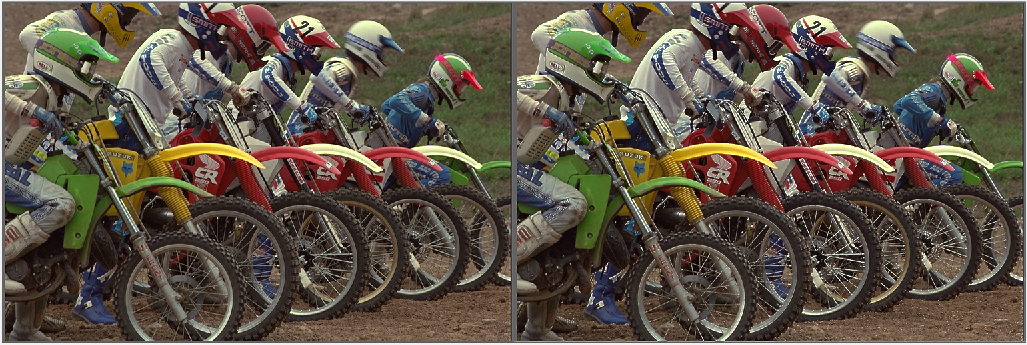C代码：
C有着matlab无法比拟的速度优势。下面是我用C写的UM代码，Srcp为输入源图像，dstp为输出的锐化后的图像，sharpen_coef为定点化后的增强系数（0-64），threshold为增强的阀值（高频分量大于该阈值，才做增强，典型值为15），低通滤波器模板为{{1,2,1},{2,4,2},{1,2,1}}的高斯核。
void imageSharpenUM(uint8_t* srcp, uint8_t* dstp, int src_width, int src_height, int src_pitch, int sharpen_coef, int threshold)
{
//int gaussianMat = {{6, 43, 6}, {43, 317, 43}, {6, 43, 6}};
int gaussianMat = {{1, 2, 1}, {2, 4, 2}, {1, 2, 1}};      // 定义低通滤波器
int dstBlur;        // 低频图像
int dstTexture;     // 细节图像
int dstDetail;      // 细节加权重
int src11 = 0;      // 11
int src12 = 0;      // 12
int src13 = 0;      // 13
int src21 = 0;      // 21
int src22 = 0;      // 22
int src23 = 0;      // 23
int src31 = 0;      // 31
int src32 = 0;      // 32
int src33 = 0;      // 33

for(int ver = 0; ver < src_height; ++ver){
for(int hor = 0; hor < src_width; ++hor){
if(ver==0 || ver==(src_height-1) || hor==0 || hor==(src_width-1)){
dstp[ver * src_pitch + hor] = srcp[ver * src_pitch + hor];
} else {
src11 = (int)srcp[(ver - 1) * src_pitch + hor - 1] * gaussianMat;
src12 = (int)srcp[(ver - 1) * src_pitch + hor] * gaussianMat;
src13 = (int)srcp[(ver - 1) * src_pitch + hor + 1] * gaussianMat;

src21 = (int)srcp[ver * src_pitch + hor - 1] * gaussianMat;
src22 = (int)srcp[ver * src_pitch + hor] * gaussianMat;
src23 = (int)srcp[ver * src_pitch + hor + 1] * gaussianMat;

src31 = (int)srcp[(ver + 1) * src_pitch + hor - 1] * gaussianMat;
src32 = (int)srcp[(ver + 1) * src_pitch + hor] * gaussianMat;
src33 = (int)srcp[(ver + 1) * src_pitch + hor + 1] * gaussianMat;

dstBlur = (src11 + src12 + src13 + src21 + src22 + src23 + src31 + src32 + src33) >> 4;
dstTexture = clip3(srcp[ver * src_pitch + hor] - dstBlur, 0, 255);
if(dstTexture > threshold) {
dstDetail = (dstTexture * sharpen_coef) >> 4;
dstp[ver * src_pitch + hor] = clip3((int)srcp[ver * src_pitch + hor] + dstDetail, 0, 255);
} else {
dstp[ver * src_pitch + hor] = srcp[ver * src_pitch + hor];
}
}
}
}
}

使用下面函数来调用sharpenUM，输入输出图像如下所示，上图是输入图像，下图是锐化后的输出图像。
imDst = sharpenUM(imSrc, 15, 2);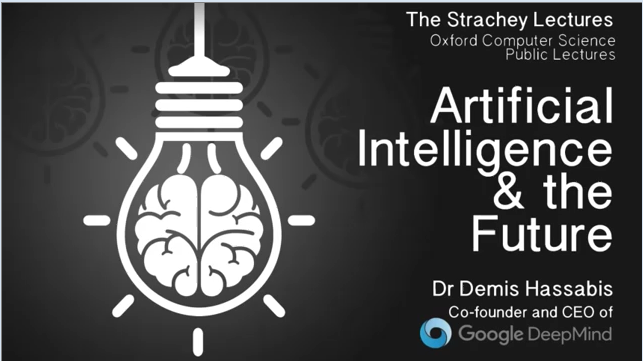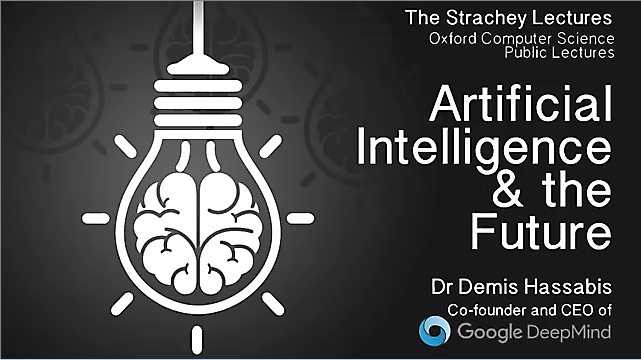硬件实现时往往使用LUT来做，根据不同的strength及threshold、偏好设计好LUT，使用高频图像进行查表，将查表的结果叠加到原图上。
参考：
 matlab -imsharpen
 数字图像处理，岗萨雷斯


展开全文matlab opencv
• 本文在掌握了图像锐化的原理，发展现状的基础上，展开研究锐化算法，提出了先用算法检出边缘,然后根据检出的边缘对图像进行高频增强的方法。介绍了一些算法，包括、LoG、Canny等算法。对常用的LoG和Canny等算子进行...
• 图像的模板运算 包括拉普拉斯锐化 梯度锐化 vc++编程得到的源代码
• 这篇文章主要给大家介绍了关于Java中图像锐化操作的相关资料，文中通过示例代码介绍的非常详细，对大家的学习或者工作具有一定的参考学习价值，需要的朋友们下面随着小编来一起学习学习吧一、该图像锐化的思想：本文...
这篇文章主要给大家介绍了关于Java中图像锐化操作的相关资料，文中通过示例代码介绍的非常详细，对大家的学习或者工作具有一定的参考学习价值，需要的朋友们下面随着小编来一起学习学习吧一、该图像锐化的思想：本文的图像锐化是将图像中的R,G,B的值分别从原图像中提出，然后将分别将这三个R,G,B的值分别与卷积核进行卷积，最终再将最后的三个卷积的结果合成为一个像素值，从而实现图像的锐化效果。二、整体的图像锐化的代码为：
package com.yf1105;

import java.awt.Color;
import java.awt.Graphics;
import java.awt.image.BufferedImage;
import java.io.File;
import java.io.IOException;

import javax.imageio.ImageIO;
import javax.swing.ImageIcon;
import javax.swing.JFrame;

public class ImageTest extends JFrame{

public static void main(String[] args) {
new ImageTest();
}
public ImageTest() {

setSize(1000,700);
setDefaultCloseOperation();
setVisible(true);

}

@Override
public void paint(Graphics g) {
(g);
int[][] rgbOfImg = getImagePixel("image/");
test(g, "锐化",rgbOfImg.length, rgbOfImg.length, rgbOfImg);
//		(new ImageIcon("img/").getImage(), 0, 0, null);
}

public void test(Graphics graphics,String text,int width,int height,int[][] rgbOfImg) {
int[][] R ,G ,B;
int size=3;
//对于不同的功能设置不同大小的矩阵
//		if(("锐化")){size = 5;}
//锐化卷积核
double[][] sharpening = {{-1,-1,-1},{-1,9,-1},{-1,-1,-1}};
//		float[][] sharpening = { { -1, -1, -1, -1, -1 }, { -1, -1, -1, -1, -1 }, { -1, -1, 25, -1, -1 },
//				{ -1, -1, -1, -1, -1 }, { -1, -1, -1, -1, -1 } };
//下面开始搞卷积算法
//初始化rgb数组
R = new int[size][size];
G = new int[size][size];
B = new int[size][size];
//对应3*3的像素格子进行卷积计算
for(int x = 0;x < width-size+1;x++){
for(int y = 0;y < height-size+1;y++){
//设置三个值分别存储r,g,b的特征值，一定要在循环内部进行初始化0，这样才能每次有不同的值
int resultOfR = 0;
int resultOfG = 0;
int resultOfB = 0;
//将格子的rgb值都取出，便于之后的卷积操作
for(int j = 0;j <size;j++){
for(int i = 0;i < size;i++){
//将该点的RGB信息取出，放到变量中待操作
int argb = rgbOfImg[x][y];

//分段获取其R,G,B信息
//int变量共4位32字节，0位对应透明度(A)，1位对应R值，2位对应G值，3位对应B值
//>>操作:将二进制代码向右移动，左边空位根据符号补充，正号为0，负号为1，右边超过范围的全部舍弃
//&:二进制位与运算符，只有两个变量对应值均为1时该位才返回1，0xff表示全为1的十六进制数(11111111),因此任何与0xff进行位与的结果均为其本身
//先移位后取位与可以将不同值对应的位信息取出,位与的意义是只取32字节的后8字节
R[i][j] = argb>>16 & 0xff;
G[i][j] = argb>>8 & 0xff;
B[i][j] = argb  & 0xff;
}
}
if(("锐化")){
//分别对R,G,B进行卷积操作，对应相乘后加起来
for(int j = 0;j < size;j++){
for(int i = 0;i < size;i++){
resultOfR += (int)(sharpening[i][j]*R[i][j]);
}
}
for(int i = 0;i < size;i++){
for(int j = 0;j < size;j++){
resultOfG += (int)(sharpening[i][j]*G[i][j]);

}
}
for(int i = 0;i < size;i++){
for(int j = 0;j < size;j++){
resultOfB += (int)(sharpening[i][j]*B[i][j]);
}
}
}
//如果超过了rgb的界限(0-255)，将其按照最大值或最小值处理
if(resultOfR > 255)resultOfR = 255;
if(resultOfR < 0)resultOfR = 0;
if(resultOfG > 255)resultOfG = 255;
if(resultOfG < 0)resultOfG = 0;
if(resultOfB > 255)resultOfB = 255;
if(resultOfB < 0)resultOfB = 0;
//根据该rgb值创建颜色对象
Color color = new Color(resultOfR, resultOfG, resultOfB);
//设置颜色，其中graphics是图像的画布(见BufferedImage类与Graphics类)
(color);
//画像素点(drawline用来画线，这里的起始与终点都是同一点，因此可以用来画像素点)
//size/2用来将像素点赋到中心元上
(x+size/2, y+size/2, x+size/2, y+size/2);
}
}

}

// 将图片数据 读到数组中
public int[][] getImagePixel(String image) {
BufferedImage bi = null;
File file = new File(image);

try {
bi = (file);
} catch (IOException e) {
// TODO Auto-generated catch block
e.printStackTrace();
}
int w = ();
int h = ();
int imgindex[][] = new int[w][h];

for (int i = 0; i < w; i++) {
for (int j = 0; j < h; j++) {
int rgb = (i, j);
imgindex[i][j] = rgb;
(" " + rgb);
}
ln();
}
return imgindex;

}
}
输出结果：虽然这个与原图的差别并不大，可能由于卷积核选择的原因。三、关键代码的解读该段代码主要是要去取出RGB图像的R，G，B值，然后分别对其R，G，B值分别进行卷积操作，然后将卷积后的结果再重新整合成一个int的像素值，从而就实现了图像的锐化处理。当时看这段代码的时候，发现这部分不是很懂，代码如下：
R[i][j] = argb>>16 & 0xff;
G[i][j] = argb>>8 & 0xff;
B[i][j] = argb  & 0xff;
不过仔细分析了一下位运算和与运算，我发现这段代码就是在分别取出R，G，B的值，因为int类的像素值中是包括了a，r，g，b四个值，int是32位的，则这四个数按顺序存在int中，每个数占8位。所以r对应得二进制需要去移动16位才能与oxff(即1111 1111)进行与运算，b对应得二进制需要去移动8位才能与oxff(即1111 1111)进行与运算，g就直接与oxff(即1111 1111)做与运算即可，虽然这样表达有点难理解，那就看下面的示意图吧：总结到此这篇关于Java中图像锐化操作的文章就介绍到这了,更多相关Java图像锐化操作内容请搜索脚本之家以前的文章或继续浏览下面的相关文章希望大家以后多多支持脚本之家！
展开全文• 图像锐化是使图像边缘更加清晰的一种图像处理方法常用的做法是提取图像的高频分量，将其叠加到原图上。 图像高频分量的提取有两种做法:一种是用高通滤波器得到高频分量；另一种是用低通滤波器得到低频，然后用原图...
基本概念
图像锐化是使图像边缘更加清晰的一种图像处理方法。常用的做法是提取图像的高频分量，将其叠加到原图上。
图像高频分量的提取有两种做法:一种是用高通滤波器得到高频分量；另一种是用低通滤波器得到低频，然后用原图减去低频得到高频。
直接提取高频的方法有sobel算法、laplcian算子，sobel算子是图像的一阶导数，提取的是梯度信息，分水平和垂直两种，常常用来做边缘检测、方向判别，sobel算子在斜坡处不为0，因此会产生较粗的边缘。laplcian算子是图像的二阶导，在图像开始变化和结束变化的地方值不为0，渐变时结果为0，因此laplacian比sobel算子更适合做sharpen。
钝化蒙版
先根据用户指定的钝化度对图像进行高斯模糊处理，再对高斯模糊处理结果与原图像进行钝化处理。 钝化度用来改变像素间的对比度强弱，钝化度，取值（0~100），钝化值越小，钝化的部分就越窄，仅仅会影响边缘像素； 钝化值越大，钝化的范围越宽，效果更明显。
// degree:钝化度，取值（0~100）
// 钝化度用来改变像素间的对比度强弱，钝化值越小，钝化的部分就越窄，仅仅会影响边缘像素
// 钝化值越大，钝化的范围越宽，效果更明显
Mat UnsharpMask(Mat src, int degree) {
int row = src.rows;
int col = src.cols;
if (degree < 1) degree = 1;
if (degree > 100) degree = 100;
Mat dst(row, col, CV_8UC3);
src.copyTo(src);
int border = 1;
for (int i = 0; i < degree; i++) {
GaussianBlur(dst, dst, Size(3, 3), 1.0);
}
for (int i = 0; i < row; i++) {
for (int j = 0; j < col; j++) {
for (int k = 0; k < 3; k++) {
int sum = 2 * src.at<Vec3b>(i, j)[k] - dst.at<Vec3b>(i, j)[k];
if (sum > 255) sum = 255;
else if (sum < 0) sum = 0;
dst.at<Vec3b>(i, j)[k] = sum;
}
}
}
return dst;
}

参考资料


展开全文• ## 图像锐化算法

千次阅读 2019-07-18 11:41:53
图像锐化，是使图像边缘更清晰的一种图像处理方法，细节增强（detail enhancement）我理解也包含了图像锐化常用的做法是提取图像的高频分量，将其叠加到原图上。图像高频分量的提取有两种做法，一种是用高通滤波器...
• 近年来，基于卷积神经网络的全色图像锐化方法取得了最先进的性能。但是现有的基于CNn的策略需要监督，低分辨率多光谱图像是简单地对高分辨率图像进行模糊和下采样得到的。另一方面，它们通常忽略全色图像丰富的空间...机器学习 深度学习 pytorch
• ## 图像锐化

千次阅读 2016-04-21 21:47:27
图像边缘分析 一阶微分算子 一梯度算子 二Robert算子 三Sobel算子 四Prewitt算子 二阶微分算子 高斯滤波和边缘检测 一高斯函数 二LOG算子 三Canny算子 频域高通滤波图像边缘分析边缘定义为图像中亮度突变的区域，...
• 图像锐化和边缘检测 本文内容构成：  1、图像锐化和边缘检测的基本概念，微分梯度已经差分的定义  2、锐化和边缘检测的像素处理方式（3种）  3、单方向一阶微分锐化，包括：  水平方向  垂直方向  ...
• 本篇将介绍图像增强范畴中的图像锐化部分，以经典的LAPLACE锐化和Photoshop USM锐化为例，通过C语言编程实现，教会大家这两种锐化算法，增强大家对图像锐化的理解以及对图像增强范畴的认知。
• 本文叙述了几种实现图像锐化的方式，包括拉普拉斯滤波，加权均值滤波，形态学梯度和顶帽底帽变换。本文的特色在于，大致证明了这几种方式可以实现图像锐化的原因。 更新记录 本文持续更新！如文中有错误，或你对...
• 图像锐化和边缘提取技术可以消除图像中的噪声，提取图像信息中用来表征图像的一些变量，为图像识别提供基础。通常使用灰度差分法对图像的边缘、轮廓进行处理，将其凸显。本文分别采用Laplacian算子、Robert算子、...Python图像处理 边缘检测 Sobel算子
• 本文档包含数字图像空间域的锐化处理，包括robert算子滤波、sobel算子滤波，以及laplace算子滤波。附代码。
• 点击上方“新机器视觉”，选择加"星标"或“置顶”重磅干货，第一时间送达本文以Dalsa sherlock软件为例，一起来了解一下视觉检测中平滑模糊的图像处理方法。1.观察灰度分布来描述一幅图像称为空间域，观察图像变化的...
• 利用Python集成了20多常用图像处理方法包括二值化、边缘检测、开闭运算、高斯模糊、直方图等操作。仅需要读取图片便能运行，可在Python3环境下直接运行，无需调整。
• ## 中值滤波与图像锐化

万次阅读 多人点赞 2016-12-24 15:47:39
图像锐化包括梯度算子、拉普拉斯算子、高提升滤波和高斯-拉普拉斯变换 本章的典型囊例分析 对椒盐噪声的平滑效果比较 Laplacian与LoG算子的锐化效果比较 中值滤波中值滤波本质上是一种统计排序滤波器． 对于原...算法
• 用MATLAB实现图像锐化的简单程序，其中包括sobel算子和拉普拉斯算子两种方法，采用调用MATLAB系统函数实现的。
• 包括几个边缘检测、去噪声、的方法，高通滤波和低通滤波等。还包括图像读取、缩放、平移等。
• 源代码是 用FPGA实现的锐化算法，适用于连续，非连续数据流，包括3x3模板的提取，空间域锐化等级的配置，视频图像宽度和高度可以任意配置。
• 本文内容构成： 1、图像锐化和边缘检测的基本概念，微分梯度已经差分的定义 2、锐化和边缘检测的像素处理方式（3种） 3、单方向一阶微分锐化，包括： 水平方向 垂直方向 Kirsch算子
• 本文内容构成： ... 1、图像锐化和边缘检测的基本概念，微分梯度已经差分的定义 2、锐化和边缘检测的像素处理方式（3种） 3、单方向一阶微分锐化，包括： 水平方向 垂直方向 Kirsch算...
• 图像锐化和边缘提取技术可以消除图像中的噪声，提取图像信息中用来表征图像的一些变量，为图像识别提供基础。通常使用灰度差分法对图像的边缘、轮廓进行处理，将其凸显。前文分别采用Laplacian算子、Robert算子、...Python图像处理 边缘检测 Canny算子 LOG算子
• 图像锐化和边缘检测 本文内容构成： 1、图像锐化和边缘检测的基本概念，微分梯度已经差分的定义 2、锐化和边缘检测的像素处理方式（3种） 3、单方向一阶微分锐化，包括： 水平方向 垂直方向 ...
• 图像处理的方法列举 1.图形灰度的集合处理：  主要有：和、并、交、补、差等。 2.逻辑操作：  图像的二值图处理时，可以有：and、not、or、and not、xor等。 3.图像配准：  用于对齐两幅或多幅相同场景的......